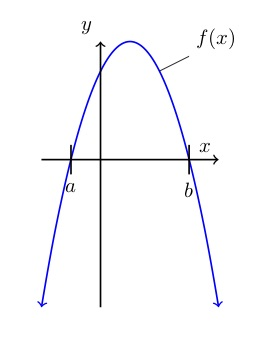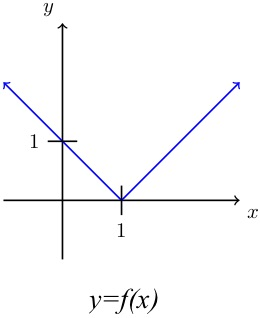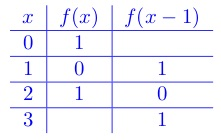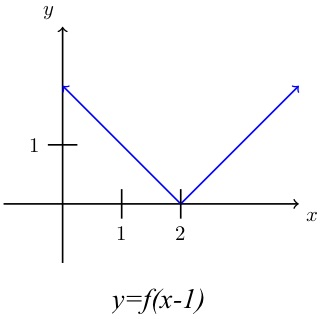# What’s up (more), the parabola or the line?

## QuestionThe graph to the left shows the function f(x), a parabola – that is, a quadratic function. Not shown on the graph is function  in which c is a negative constant.

One statement below is true. Which one?

## Solution

What’s going on? The line will cross the parabola in a couple of places and those intersections will determine which function is higher in various places. But all you know about function is that  and that c is negative.

is the equation of a straight line. Think of a line’s basic equation:  where b is the line’s y-intercept – that is, y’s value where . In this case, because  nothing, the y-intercept is 0. That means the line goes through the origin.

Also, you’re told that c is negative. c is in the role of m in the equation that is, it’s the slope. The negative slope means this line goes down as it goes to the right.

How steep is the line? You are given no information about that. Take a guess.

The brown line in the graph to the right shows what function g may look like. It may be steeper or less steep than shown,but it will cross the x-axis at the origin, and it will go down as it goes to the right, as shown.

Let’s go through all the possible answers to find the one true statement.

1. . At , as you can see from the graph, the parabola is above the x-axis, which means f(0) is a positive value. The line goes right through the origin – so . Thus which means it cannot be true that .
2. . At , function f crosses the x-axis. So . At , function g crosses the x-axis. So . That means f(a) is equal to g(0), so it’s not possible that f(a) is less than g(0).
3. . What’s happening at ? Function f crosses the x-axis there, so ; and function g has a positive value. That means g is more than f, so it must be that . Looks like that’s the answer, but let’s still check the others.
4. . We have established that . And since g(x) goes through the origin and slopes down, g must be less than zero at any x-value that is greater than 0 – like, for example, b. That means g is less than f, so it is not possible that f is less than g.
5. . As you can see from the graph, . And, as we said in response to answer #4, g(b) is less than 0. So f(b) must be more than g(b) – and it’s not possible that .

I found this question intimidating, because it seems so difficult to know anything about function g. But once you look closely, it’s not so bad. The key is to notice that g takes on positive values to the left of and negative values to the right of .

# Horizontal Transformation: Sliding a Function Sideways

## Question

This is the graph ofSketch the graph of .

## Solution

The change from  to  is called a horizontal transformation; it slides the function sideways. The graph of  lies one unit to the right of the graph of . This may be counterintuitive, so let’s look at some numbers. At each x-value, the graph of  takes the y-value that the graph of  takes one unit earlier. Some values on the two graphs look like this:The y-values from the original graph have all chugged along to correspond to higher x-values than they did before.At any x-value on the graph, the function  operates on the  value 1 unit to the left of that x-value. That has the effect of moving the curve 1 unit to the right.

Similarly,  graphs one unit to the left of . Note that this is different from  which graphs one unit up from  because the 1 that gets added to the function means 1 gets added to every y-value. Similarly,  graphs one unit below  If you don’t believe me on any of these, try playing around with values of a simple function to see what happens.